# Coordinates of a centroind

Let’s A = [3, 2, 0], B = [1, -2, 4] and C = [1, 1, 1] be 3 points in space. Calculate the coordinates of the centroid of △ABC (the intersection of the medians).

x =  1.6667
y =  0.3333
z =  1.6667

### Step-by-step explanation:

$y=\frac{{y}_{0}+{y}_{1}+{y}_{2}}{3}=\frac{2+\left(-2\right)+1}{3}=\frac{1}{3}=0.3333$
$z=\frac{{z}_{0}+{z}_{1}+{z}_{2}}{3}=\frac{0+4+1}{3}=\frac{5}{3}=1\frac{2}{3}=1.6667$

Try calculation via our triangle calculator.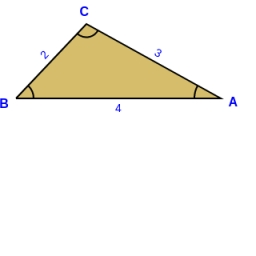Did you find an error or inaccuracy? Feel free to write us. Thank you!Tips to related online calculators
Looking for help with calculating arithmetic mean?
Line slope calculator is helpful for basic calculations in analytic geometry. The coordinates of two points in the plane calculate slope, normal and parametric line equation(s), slope, directional angle, direction vector, the length of the segment, intersections of the coordinate axes, etc.
Looking for a statistical calculator?
Our vector sum calculator can add two vectors given by their magnitudes and by included angle.

#### You need to know the following knowledge to solve this word math problem:

We encourage you to watch this tutorial video on this math problem:

## Related math problems and questions:

• Medians and sidesTriangle ABC in the plane Oxy; are the coordinates of the points: A = 2.7 B = -4.3 C-6-1 Try calculate lengths of all medians and all sides.
• Three points 2The three points A(3, 8), B(6, 2) and C(10, 2). The point D is such that the line DA is perpendicular to AB, and DC is parallel to AB. Calculate the coordinates of D.
• Triangle's centroid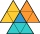In the triangle ABC the given lengths of its medians tc = 9, ta = 6. Let T be the intersection of the medians (triangle's centroid) and point is S the center of the side BC. The magnitude of the CTS angle is 60°. Calculate the length of the BC side to 2 d
• Three points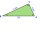Three points K (-3; 2), L (-1; 4), M (3, -4) are given. Find out: (a) whether the triangle KLM is right b) calculate the length of the line to the k side c) write the coordinates of the vector LM d) write the directional form of the KM side e) write the d
• CoordinatesDetermine the coordinates of the vertices and the content of the parallelogram, the two sides of which lie on the lines 8x + 3y + 1 = 0, 2x + y-1 = 0 and the diagonal on the line 3x + 2y + 3 = 0
• Vector - basic operationsThere are given points A [-9; -2] B [2; 16] C [16; -2] and D [12; 18] a. Determine the coordinates of the vectors u=AB v=CD s=DB b. Calculate the sum of the vectors u + v c. Calculate difference of vectors u-v d. Determine the coordinates of the vector w
• LineStraight-line passing through points A [-3; 22] and B [33; -2]. Determine the total number of points of the line in which both coordinates are positive integers.
• Calculate 6Calculate the distance of a point A[0, 2] from a line passing through points B[9, 5] and C[1, -1].
• Curve and lineThe equation of a curve C is y=2x² -8x+9, and the equation of a line L is x+ y=3 (1) Find the x coordinates of the points of intersection of L and C. (2) Show that one of these points is also the stationary point of C?
• Coordinates hexagon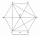The regular hexagon ABCDEF is given. Point A has coordinates [1; 3], and point D has coordinates [4; 7]. Calculate the sum of the coordinates of the center of its described circle.
• Center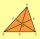In the triangle ABC is point D[1,-2,6], which is the center of the |BC| and point G[8,1,-3], which is the center of gravity of the triangle. Find the coordinates of the vertex A[x,y,z].
• The triangleThe triangle is given by three vertices: A [0.0] B [-4.2] C [-6.0] Calculate V (intersection of heights), T (center of gravity), O - center of a circle circumscribed
• Triangle IRTAn isosceles right triangle ABC with right angle at vertex C has vertex coordinates: A (-1, 2); C (-5, -2) Calculate the length of segment AB.
• Segment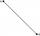Calculate the segment AB's length if the coordinates of the end vertices are A[10, -4] and B[5, 5].
• Center of line segmentCalculate the distance of the point X [1,3] from the center of the line segment x = 2-6t, y = 1-4t ; t is from interval <0,1>.
• Center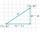Calculate the coordinates of the center of gravity T [x, y] of triangle ABC; A[11,4] B[13,-7] C[-17,-18].
• Line intersect segment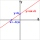Decide whether the line p : x + 2 y - 7 = 0 intersects the line segment given by points A[1, 1] and B[5, 3]